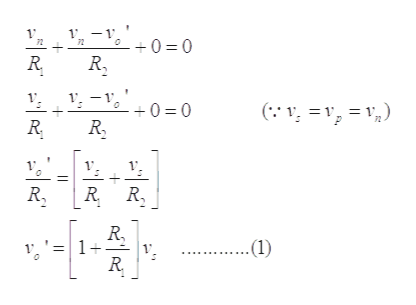# Some of the exercises show op-amps with a positive supply voltage but no information about negative supply voltages. Whenever this is encountered, assume the negative supply voltage has the same magnitude as that of the positive supply

Question
1 views
Some of the exercises show op-amps with a positive supply voltage but no information about negative supply voltages. Whenever this is encountered, assume the negative supply voltage has the same magnitude as that of the positive supply
check_circle

Step 1

Mark the current and node voltages in given circuit as shown below:

Step 2

For ideal-op-amp, the current in inverting and non-inverting terminal are zero, due to the high input resistance of op-amp.

By virtual ground concept, the voltage at the inverting terminal should be equal to the non-inverting terminal.

Step 3

Applying the nodal analysis a...help_outlineImage Transcriptionclose1 1 1 2 0 0 1 0 0 R2 = 1 R R. R, 1+ .(1) fullscreen

### Want to see the full answer?

See Solution

#### Want to see this answer and more?

Solutions are written by subject experts who are available 24/7. Questions are typically answered within 1 hour.*

See Solution
*Response times may vary by subject and question.
Tagged in

### Electrical Engineering